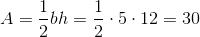# SSAT Upper Level Math : How to find the area of a right triangle

## Example Questions

2 Next →

### Example Question #11 : How To Find The Area Of A Right Triangle

What is the area of a right triangle whose hypotenuse is 13 inches and whose legs each measure a number of inches equal to an integer?

It cannot be determined from the information given.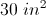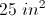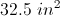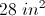We are looking for a Pythagorean triple - that is, three integers that satisfy the relationship. We know that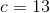, and the only Pythagorean triple withis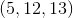. The legs of the triangle are therefore 5 and 12, and the area of the right triangle is